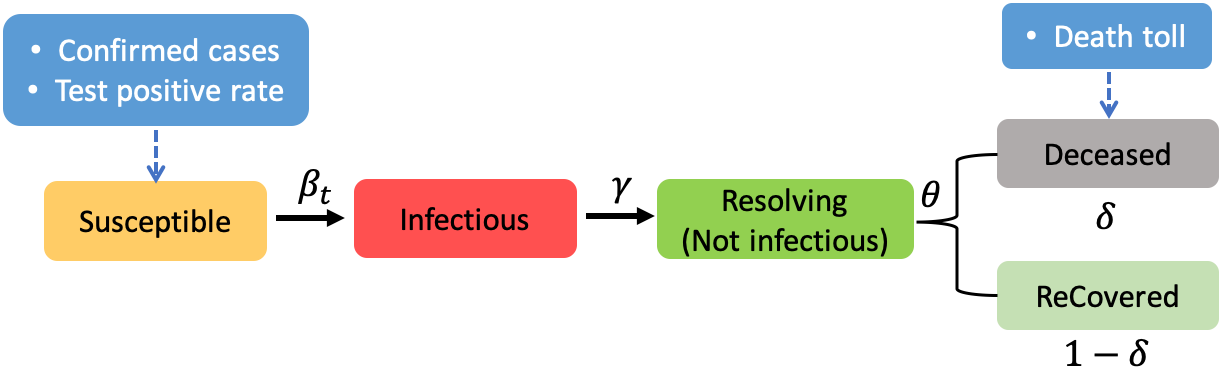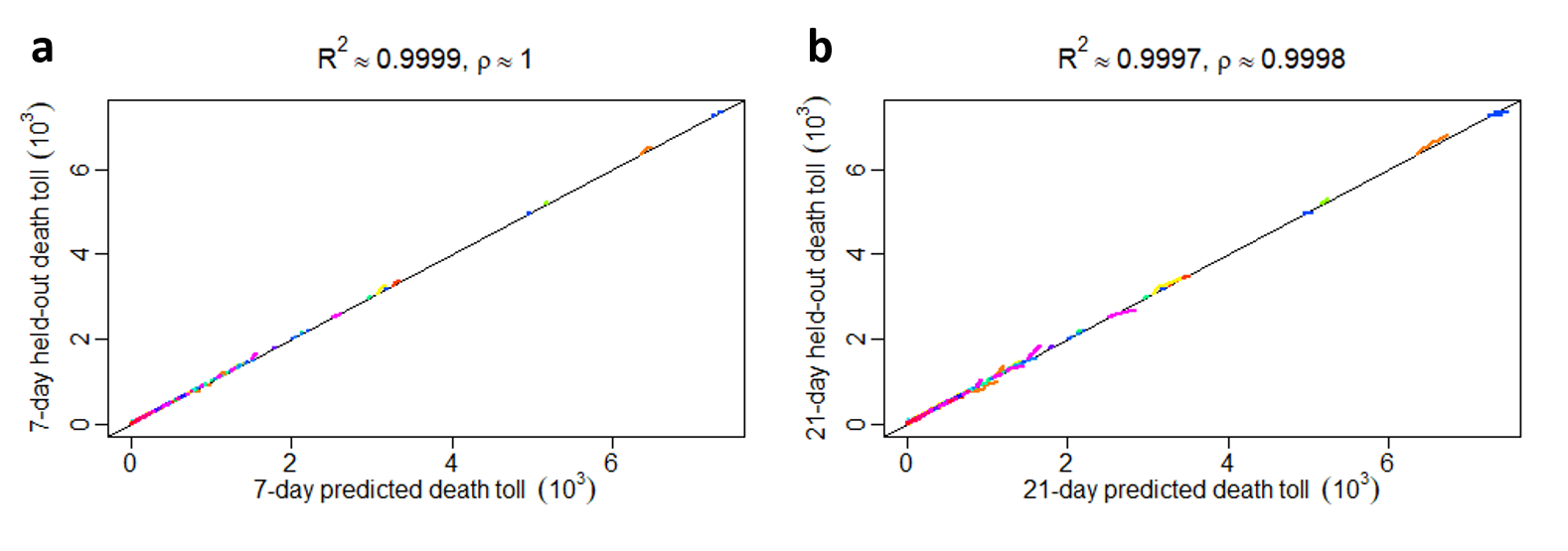### Daily Probability of Contracting (PoC) COVID-19 in the U.S.

Select U.S. National Map in Map range to generate the national PoC COVID-19 map. It may take 10~15 seconds.

Note: the daily PoC COVID-19 measures the average daily probability to contract COVID-19 for a susceptible individual in a community.

Note: if the videos aren't playing well, please make sure you are using the latest version of the browser (Chrome is highly recommended).
Note: the effective reproduction number, $R_{eff}$, is defined as the average number of secondary cases per primary case on a certain date. The effective reproduction number at date $t$ can be computed by $R_{eff}(t)=R_0(t) S(t)/N$, where $R_0(t)$ and $S(t)$ denote the basic reproduction number and susceptible population size at date $t$, respectively, and $N$ is the total population size.
Note: The figure shows the estimated number of infectious individuals who can transmit COVID-19 to others in this area on a certain date. These infectious individuals are often not diagnosed yet.

We developed a robust algorithm to integrate testing data and death toll to monitor and forecast the COVID-19 transmission characteristic in all 3,048 counties of US counties with no less than 2 cumulative deaths as of July 22, 2021 and beyond. We found that slightly reducing the transmission period of SARS-CoV-2 can substantially reduce the COVID-19 associated deaths; expanding diagnostic tests along with other mitigation measures can achieve this goal .

### Data

The datasets used in our model are listed as below:

### Model DescriptionFigure 1. SIRDC model. The blue rectangle denotes the data used in the county level estimation.

Let us consider a modified SIR model (in case you are not familiar with SIR model, check Wikipedia or reference ), named the SIRDC model , which models the transmission dynamics of COVID-19 by 5 compartments: susceptible ($$S$$), Infective ($$I$$), Resolving ($$R$$), Deceased ($$D$$) and reCovered ($$C$$). At the beginning of the epidemic, almost all individuals in a community are susceptible. Then, as illustrated by Figure 1, part of the population are infected by the active infectious individuals, and the time-dependent transmission rate is denoted by $$\beta_t$$. After $$\frac{1}{\gamma}$$ days, the infectious individuals are expected to become not infectious (denoted as resolving compartment), meaning that they will not transmit COVID-19 to others due to hospitalization or self-quarantine. After $$\frac{1}{\theta}$$ days, a resolving case will be resolved (either recovered or deceased). The proportion of deaths from the number of resolved cases is controlled by the fatality rate parameter $$\delta$$.

For convenience, we list the interpretations of the math symbol below:

• $$S(t)$$: the number of susceptible cases at date $$t$$. A susceptible case is defined the individual who does not have antibodies of COVID-19.
• $$I(t)$$: the number of infectious cases at date $$t$$. An infectious case is defined as the individual who can transmit COVID-19 to others.
• $$R(t)$$: the number of resolving cases at date $$t$$. A resolving case is defined as the individual who is unable to transmit it to others due to hospitalization or self-quarantine, but has not resolved yet.
• $$D(t)$$: the number of total deaths at date $$t$$.
• $$C(t)$$: the number of total recovered cases at date $$t$$.

The SIRDC model for the county $$j$$ in the state $$i$$ in US is described by the following 5 ordinary differential equations:

$\dot{S}_{i,j}(t)= \frac{-\beta_{i,j}(t) S_{i,j}(t) I_{i,j}(t)}{N_{i,j}}$

$\dot{I}_{i,j}(t)=\frac{\beta_{i,j}(t) S_{i,j}(t) I_{i,j}(t)}{ N_{i,j}}-{\gamma I_{i,j}(t)}$ $\dot{R}_{i,j}(t)= {\gamma I_{i,j}(t)}-{\theta R_{i,j}(t)}$

$\dot{D}_{i,j}(t)={\delta \theta R_{i,j}(t)}$

$\dot{C}_{i,j}(t)={(1-\delta) \theta R_{i,j}(t)}$

where $$N_{i,j}$$ stands for the number of population in that region.

The following are some brief introductions for the terminologies that describe the transmission dynamic of COVID-19.

• Daily probability of contracting (PoC) COVID-19: the daily PoC COVID-19 measures the average probability to contract COVID-19 for a susceptible individual in a community on a certain day. The daily PoC COVID-19 on day $$t$$ is defined as $${\beta_{i,j}(t)I_{i,j}(t)}/{N_{i,j}}$$.

• Effective reproduction number: the effective reproduction number, $$R_{eff}$$, is defined as the average number of secondary cases per primary case on a certain date. The effective reproduction number in a certain area on day $$t$$ can be computed by $$R_{eff}^{i,j}(t)= \frac{\beta_{i,j}(t)}{\gamma} \frac{S_{i,j}(t)}{N_{i,j}}$$, where $$\frac{\beta_{i,j}(t)}{\gamma}$$ denotes the basic reproduction number in this area on day $$t$$.

• Active infectious individuals: the active infectious individuals, $$I_{i,j}(t)$$, is the number of individuals who can transmit COVID-19 to others in this area on day $$t$$. These infectious individuals are often not diagnosed yet.

The innovation of our approach is on solving the compartmental models using a midpoint rule with a step size of 1 day as the confirmed cases and death toll are updated daily in most US counties. Our estimate of transmission rates and reproduction numbers is robust and accurate to reproduce the number of death toll and other compartments for counties with medium to small population sizes.

### Validation

How do we know our model works well? We evaluate our model based on forecasting the future deaths for all counties we considered. We graph the 7-day and 21-day forecasts for all counties with no less than 2 deaths using data as of Sep 20, 2020 in Figure 2 below.Figure 2. 7-day and 21-day forecasts for all counties with no less than 2 deaths by the SIRDC model. The same color represents the counties from the same state.

### Surveillance

The number of active infectious individuals and time-dependent effective reproduction rate are important to determine the mitigation policy at the county level. Note that a significant number of COVID-19 patients are asymptomatic and they may not be diagnosed [2,4]. Estimating the number of active infectious individuals (who can transmit the COVID-19 in a community but are not diagnosed) is thus important for mitigating the COVID-19 transmission. More diagnostic tests and nonpharmaceutical interventions should be considered when the PoC COVID-19, the estimated number of active infectious individuals or the effective reproduction rate significantly increase in a community.

### References

 Li, H. and Gu, M. (2020). Robust estimation of SARS-CoV-2 epidemic at US counties. arXiv preprint arXiv:2010.11514.

 Anand, Shuchi, et al. Prevalence of SARS-CoV-2 antibodies in a large nationwide sample of patients on dialysis in the USA: a cross-sectional study. Lancet (2020).

 Fernández-Villaverde, Jesús, and Charles I. Jones. Estimating and Simulating a SIRD Model of COVID-19 for Many Countries, States, and Cities. No. w27128. National Bureau of Economic Research (2020).

 Havers, Fiona P., et al. Seroprevalence of antibodies to SARS-CoV-2 in 10 sites in the United States, March 23-May 12, 2020. JAMA Internal Medicine (2020).

 Hethcote, Herbert W. The mathematics of infectious diseases. SIAM review 42.4 (2000): 599-653.

## Maintainers:

• Dr. Mengyang Gu , Assistant Professor in PSTAT, UCSB, Email: mengyang at pstat dot ucsb dot edu
• Hanmo Li, PhD Student in PSTAT, UCSB
• Siqi He, Undergraduate Student in PSTAT, UCSB
• Patrick Windmiller, IT staff, UCSB

## Acknowledgements

Research reported on this website was supported by the National Institute Of Diabetes And Digestive And Kidney Diseases of the National Institutes of Health under Award Number R01DK130067. The content is solely the responsibility of the authors and does not necessarily represent the official views of the National Institutes of Health.